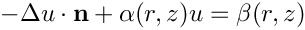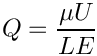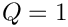oomph::DiskLikeGeomObjectWithBoundaries Class Reference

Base class for upgraded disk-like GeomObject (i.e. 2D surface in 3D space) with specification of boundaries. The GeomObject's position(...) function computes the 3D (Eulerian) position vector r as a function of the 2D intrinsic (Lagrangian) coordinates, zeta, without reference to any boundaries. This class specifies the boundaries by specifying a mapping from a 1D intrinsic boundary coordinate, zeta_bound, to the 2D intrinsic (Lagrangian) coordinates, zeta. More...

#include <geom_obj_with_boundary.h>Inheritance diagram for oomph::DiskLikeGeomObjectWithBoundaries:

## Public Member Functions

DiskLikeGeomObjectWithBoundaries ()
Constructor. More...

unsigned nboundary () const
How many boundaries do we have? More...

void position_on_boundary (const unsigned &b, const double &zeta_bound, Vector< double > &r) const
Compute 3D vector of Eulerian coordinates at 1D boundary coordinate zeta_bound on boundary b: More...

void zeta_on_boundary (const unsigned &b, const double &zeta_bound, Vector< double > &zeta) const
Compute 2D vector of intrinsic coordinates at 1D boundary coordinate zeta_bound on boundary b: More...

GeomObjectboundary_parametrising_geom_object_pt (const unsigned &b) const
Pointer to GeomObject<1,2> that parametrises intrinisc coordinates along boundary b. More...

double zeta_boundary_start (const unsigned &b) const
Initial value of 1D boundary coordinate zeta_bound on boundary b: More...

double zeta_boundary_end (const unsigned &b) const
Final value of 1D boundary coordinate zeta_bound on boundary b: More...

virtual void boundary_triad (const unsigned &b, const double &zeta_bound, Vector< double > &r, Vector< double > &tangent, Vector< double > &normal, Vector< double > &binormal)
Boundary triad on boundary b at boundary coordinate zeta_bound. Broken virtual. More...

void output_boundaries (const unsigned &nplot, std::ofstream &two_d_boundaries_file, std::ofstream &three_d_boundaries_file)
Output boundaries at nplot plot points. Streams: More...

void output_boundaries_and_triads (const unsigned &nplot, std::ofstream &two_d_boundaries_file, std::ofstream &three_d_boundaries_file, std::ofstream &boundaries_tangent_file, std::ofstream &boundaries_normal_file, std::ofstream &boundaries_binormal_file)
Output boundaries and triad at nplot plot points. Streams: More...

void add_region_coordinates (const unsigned &r, Vector< double > &zeta_in_region)
Specify intrinsic coordinates of a point within a specified region – region ID, r, should be positive. More...

std::map< unsigned, Vector< double > > zeta_in_region () const
Return map that stores zeta coordinates of points that identify regions. More...Public Member Functions inherited from oomph::GeomObject
GeomObject ()
Default constructor. More...

GeomObject (const unsigned &ndim)
Constructor: Pass dimension of geometric object (# of Eulerian coords = # of Lagrangian coords; no time history available/needed) More...

GeomObject (const unsigned &nlagrangian, const unsigned &ndim)
Constructor: pass # of Eulerian and Lagrangian coordinates. No time history available/needed. More...

GeomObject (const unsigned &nlagrangian, const unsigned &ndim, TimeStepper *time_stepper_pt)
Constructor: pass # of Eulerian and Lagrangian coordinates and pointer to time-stepper which is used to handle the position at previous timesteps and allows the evaluation of veloc/acceleration etc. in cases where the GeomData varies with time. More...

GeomObject (const GeomObject &dummy)=delete
Broken copy constructor. More...

void operator= (const GeomObject &)=delete
Broken assignment operator. More...

virtual ~GeomObject ()
(Empty) destructor More...

unsigned nlagrangian () const
Access function to # of Lagrangian coordinates. More...

unsigned ndim () const
Access function to # of Eulerian coordinates. More...

void set_nlagrangian_and_ndim (const unsigned &n_lagrangian, const unsigned &n_dim)
Set # of Lagrangian and Eulerian coordinates. More...

TimeStepper *& time_stepper_pt ()
Access function for pointer to time stepper: Null if object is not time-dependent. More...

TimeSteppertime_stepper_pt () const
Access function for pointer to time stepper: Null if object is not time-dependent. Const version. More...

virtual unsigned ngeom_data () const
How many items of Data does the shape of the object depend on? This is implemented as a broken virtual function. You must overload this for GeomObjects that contain geometric Data, i.e. GeomObjects whose shape depends on Data that may contain unknowns in the overall Problem. More...

virtual Datageom_data_pt (const unsigned &j)
Return pointer to the j-th Data item that the object's shape depends on. This is implemented as a broken virtual function. You must overload this for GeomObjects that contain geometric Data, i.e. GeomObjects whose shape depends on Data that may contain unknowns in the overall Problem. More...

virtual void position (const Vector< double > &zeta, Vector< double > &r) const =0
Parametrised position on object at current time: r(zeta). More...

virtual void position (const unsigned &t, const Vector< double > &zeta, Vector< double > &r) const
Parametrised position on object: r(zeta). Evaluated at previous timestep. t=0: current time; t>0: previous timestep. Works for t=0 but needs to be overloaded if genuine time-dependence is required. More...

virtual void position (const double &t, const Vector< double > &zeta, Vector< double > &r) const
Parametrised position on object: r(zeta). Evaluated at the continuous time value, t. More...

virtual void dposition_dt (const Vector< double > &zeta, const unsigned &j, Vector< double > &drdt)
j-th time-derivative on object at current time:. More...

virtual void dposition (const Vector< double > &zeta, DenseMatrix< double > &drdzeta) const
Derivative of position Vector w.r.t. to coordinates:= drdzeta(alpha,i). Evaluated at current time. More...

virtual void d2position (const Vector< double > &zeta, RankThreeTensor< double > &ddrdzeta) const
2nd derivative of position Vector w.r.t. to coordinates:= ddrdzeta(alpha,beta,i). Evaluated at current time. More...

virtual void d2position (const Vector< double > &zeta, Vector< double > &r, DenseMatrix< double > &drdzeta, RankThreeTensor< double > &ddrdzeta) const
Posn Vector and its 1st & 2nd derivatives w.r.t. to coordinates:= drdzeta(alpha,i).= ddrdzeta(alpha,beta,i). Evaluated at current time. More...

virtual void locate_zeta (const Vector< double > &zeta, GeomObject *&sub_geom_object_pt, Vector< double > &s, const bool &use_coordinate_as_initial_guess=false)
A geometric object may be composed of may sub-objects (e.g. a finite-element representation of a boundary). In order to implement sparse update functions, it is necessary to know the sub-object and local coordinate within that sub-object at a given intrinsic coordinate, zeta. Note that only one sub-object can "cover" any given intrinsic position. If the position is at an "interface" between sub-objects, either one can be returned. The default implementation merely returns, the pointer to the "entire" GeomObject and the coordinate, zeta The optional boolean flag only applies if a Newton method is used to find the value of zeta, and if true the value of the coordinate s is used as the initial guess for the method. If the flag is false (the default) a value of s=0 is used as the initial guess. More...

virtual void interpolated_zeta (const Vector< double > &s, Vector< double > &zeta) const
A geometric object may be composed of many sub-objects each with their own local coordinate. This function returns the "global" intrinsic coordinate zeta (within the compound object), at a given local coordinate s (i.e. the intrinsic coordinate of the sub-GeomObject. In simple (non-compound) GeomObjects, the local intrinsic coordinate is the global intrinsic coordinate and so the function merely returns s. To make it less likely that the default implementation is called in error (because it is not overloaded in a derived GeomObject where the default is not appropriate, we do at least check that s and zeta have the same size if called in PARANOID mode. More...

## Protected Attributes

Vector< double > Zeta_boundary_start
Storage for initial value of 1D boundary coordinate on boundary b: More...

Vector< double > Zeta_boundary_end
Storage for final value of 1D boundary coordinate on boundary b: More...

Vector< GeomObject * > Boundary_parametrising_geom_object_pt
Pointer to GeomObject<1,2> that parametrises intrinisc coordinates along boundary b; essentially provides a wrapper to zeta_on_boundary(...) More...

std::map< unsigned, Vector< double > > Zeta_in_region
Map to store zeta coordinates of points that identify regions. More...Protected Attributes inherited from oomph::GeomObject
unsigned NLagrangian
Number of Lagrangian (intrinsic) coordinates. More...

unsigned Ndim
Number of Eulerian coordinates. More...

TimeStepperGeom_object_time_stepper_pt

## Detailed Description

Base class for upgraded disk-like GeomObject (i.e. 2D surface in 3D space) with specification of boundaries. The GeomObject's position(...) function computes the 3D (Eulerian) position vector r as a function of the 2D intrinsic (Lagrangian) coordinates, zeta, without reference to any boundaries. This class specifies the boundaries by specifying a mapping from a 1D intrinsic boundary coordinate, zeta_bound, to the 2D intrinsic (Lagrangian) coordinates, zeta.

The class is made functional by provision (in the derived class!) of:

• a pointer to a GeomObject<1,2> that parametrises the 2D intrinisic coordinates zeta along boundary b in terms of the 1D boundary coordinate, zeta_bound,
• the initial value of the 1D boundary coordinate zeta_bound on boundary b,
• the final value of the 1D boundary coordinate zeta_bound on boundary b

for each of these boundaries. All three containers for these must be resized (consistently) and populated in the derived class. Number of boundaries is inferred from their size.

Class also provides broken virtual function to specify boundary triads, and various output functions.

Definition at line 66 of file geom_obj_with_boundary.h.

## ◆ DiskLikeGeomObjectWithBoundaries()

 oomph::DiskLikeGeomObjectWithBoundaries::DiskLikeGeomObjectWithBoundaries ( )
inline

Constructor.

Definition at line 70 of file geom_obj_with_boundary.h.

## Member Function Documentation

 void oomph::DiskLikeGeomObjectWithBoundaries::add_region_coordinates ( const unsigned & r, Vector< double > & zeta_in_region )
inline

Specify intrinsic coordinates of a point within a specified region – region ID, r, should be positive.

Definition at line 256 of file geom_obj_with_boundary.h.

References zeta_in_region(), and Zeta_in_region.

## ◆ boundary_parametrising_geom_object_pt()

 GeomObject * oomph::DiskLikeGeomObjectWithBoundaries::boundary_parametrising_geom_object_pt ( const unsigned & b ) const
inline

Pointer to GeomObject<1,2> that parametrises intrinisc coordinates along boundary b.

Definition at line 137 of file geom_obj_with_boundary.h.

References Boundary_parametrising_geom_object_pt.

 virtual void oomph::DiskLikeGeomObjectWithBoundaries::boundary_triad ( const unsigned & b, const double & zeta_bound, Vector< double > & r, Vector< double > & tangent, Vector< double > & normal, Vector< double > & binormal )
inlinevirtual

Boundary triad on boundary b at boundary coordinate zeta_bound. Broken virtual.

Reimplemented in oomph::WarpedCircularDisk.

Definition at line 159 of file geom_obj_with_boundary.h.

## ◆ nboundary()

 unsigned oomph::DiskLikeGeomObjectWithBoundaries::nboundary ( ) const
inline

How many boundaries do we have?

Definition at line 73 of file geom_obj_with_boundary.h.

References Boundary_parametrising_geom_object_pt.

## ◆ output_boundaries()

 void oomph::DiskLikeGeomObjectWithBoundaries::output_boundaries ( const unsigned & nplot, std::ofstream & two_d_boundaries_file, std::ofstream & three_d_boundaries_file )
inline

Output boundaries at nplot plot points. Streams:

• two_d_boundaries_file: zeta_0, zeta_1, zeta_bound
• three_d_boundaries_file : x, y, z, zeta_0, zeta_1, zeta_bound

Definition at line 176 of file geom_obj_with_boundary.h.

 void oomph::DiskLikeGeomObjectWithBoundaries::output_boundaries_and_triads ( const unsigned & nplot, std::ofstream & two_d_boundaries_file, std::ofstream & three_d_boundaries_file, std::ofstream & boundaries_tangent_file, std::ofstream & boundaries_normal_file, std::ofstream & boundaries_binormal_file )
inline

Output boundaries and triad at nplot plot points. Streams:

• two_d_boundaries_file: zeta_0, zeta_1, zeta_bound
• three_d_boundaries_file : x, y, z, zeta_0, zeta_1, zeta_bound
• boundaries_tangent_file : x, y, z, t_x, t_y, t_z
• boundaries_normal_file : x, y, z, n_x, n_y, n_z
• boundaries_binormal_file: x, y, z, N_x, N_y, N_z

Definition at line 198 of file geom_obj_with_boundary.h.

Referenced by output_boundaries().

## ◆ position_on_boundary()

 void oomph::DiskLikeGeomObjectWithBoundaries::position_on_boundary ( const unsigned & b, const double & zeta_bound, Vector< double > & r ) const
inline

Compute 3D vector of Eulerian coordinates at 1D boundary coordinate zeta_bound on boundary b:

Definition at line 80 of file geom_obj_with_boundary.h.

References oomph::GeomObject::position(), and zeta_on_boundary().

## ◆ zeta_boundary_end()

 double oomph::DiskLikeGeomObjectWithBoundaries::zeta_boundary_end ( const unsigned & b ) const
inline

Final value of 1D boundary coordinate zeta_bound on boundary b:

Definition at line 151 of file geom_obj_with_boundary.h.

References Zeta_boundary_end.

## ◆ zeta_boundary_start()

 double oomph::DiskLikeGeomObjectWithBoundaries::zeta_boundary_start ( const unsigned & b ) const
inline

Initial value of 1D boundary coordinate zeta_bound on boundary b:

Definition at line 144 of file geom_obj_with_boundary.h.

References Zeta_boundary_start.

## ◆ zeta_in_region()

 std::map< unsigned, Vector< double > > oomph::DiskLikeGeomObjectWithBoundaries::zeta_in_region ( ) const
inline

Return map that stores zeta coordinates of points that identify regions.

Definition at line 307 of file geom_obj_with_boundary.h.

References Zeta_in_region.

## ◆ zeta_on_boundary()

 void oomph::DiskLikeGeomObjectWithBoundaries::zeta_on_boundary ( const unsigned & b, const double & zeta_bound, Vector< double > & zeta ) const
inline

Compute 2D vector of intrinsic coordinates at 1D boundary coordinate zeta_bound on boundary b:

Definition at line 91 of file geom_obj_with_boundary.h.

## ◆ Boundary_parametrising_geom_object_pt

 Vector oomph::DiskLikeGeomObjectWithBoundaries::Boundary_parametrising_geom_object_pt
protected

Pointer to GeomObject<1,2> that parametrises intrinisc coordinates along boundary b; essentially provides a wrapper to zeta_on_boundary(...)

Definition at line 324 of file geom_obj_with_boundary.h.

## ◆ Zeta_boundary_end

 Vector oomph::DiskLikeGeomObjectWithBoundaries::Zeta_boundary_end
protected

Storage for final value of 1D boundary coordinate on boundary b:

Definition at line 319 of file geom_obj_with_boundary.h.

## ◆ Zeta_boundary_start

 Vector oomph::DiskLikeGeomObjectWithBoundaries::Zeta_boundary_start
protected

Storage for initial value of 1D boundary coordinate on boundary b:

Definition at line 315 of file geom_obj_with_boundary.h.

## ◆ Zeta_in_region

 std::map > oomph::DiskLikeGeomObjectWithBoundaries::Zeta_in_region
protected

Map to store zeta coordinates of points that identify regions.

Definition at line 327 of file geom_obj_with_boundary.h.